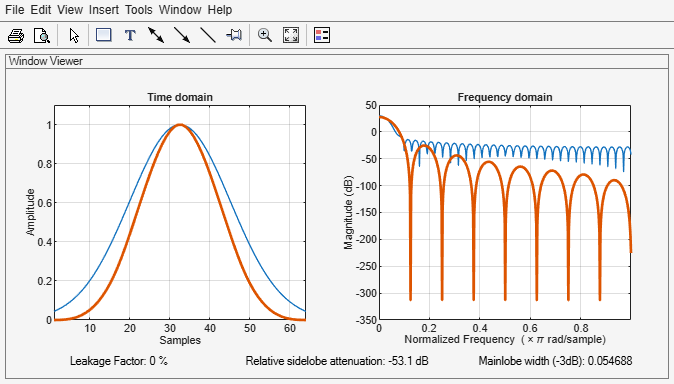# parzenwin

Parzen (de la Vallée Poussin) window

## Syntax

```w = parzenwin(L) ```

## Description

`w = parzenwin(L)` returns the `L`-point Parzen (de la Vallée Poussin) window in a column vector, `w`. Parzen windows are piecewise-cubic approximations of Gaussian windows. Parzen window sidelobes fall off as 1/ω4. See Algorithms for the equation that defines the Parzen window.

## Examples

collapse all

Compare 64-point Parzen and Gaussian windows. Display the result using `wvtool`.

```gw = gausswin(64); pw = parzenwin(64); wvtool(gw,pw)```## Algorithms

The following equation defines the `N`–point Parzen window over the interval $-\frac{\left(N-1\right)}{2}\le n\le \frac{\left(N-1\right)}{2}$:

`$w\left(n\right)=\left\{\begin{array}{cc}1-6{\left(\frac{|n|}{N/2}\right)}^{2}+6{\left(\frac{|n|}{N/2}\right)}^{3}& 0\le |n|\le \left(N-1\right)/4\\ 2{\left(1-\frac{|n|}{N/2}\right)}^{3}& \left(N-1\right)/4<|n|\le \left(N-1\right)/2\end{array}$`

## References

 harris, fredric j. "On the Use of Windows for Harmonic Analysis with the Discrete Fourier Transform." Proceedings of the IEEE®. Vol. 66, January 1978, pp. 51–83.

## Extended Capabilities

### C/C++ Code GenerationGenerate C and C++ code using MATLAB® Coder™.

Introduced before R2006a